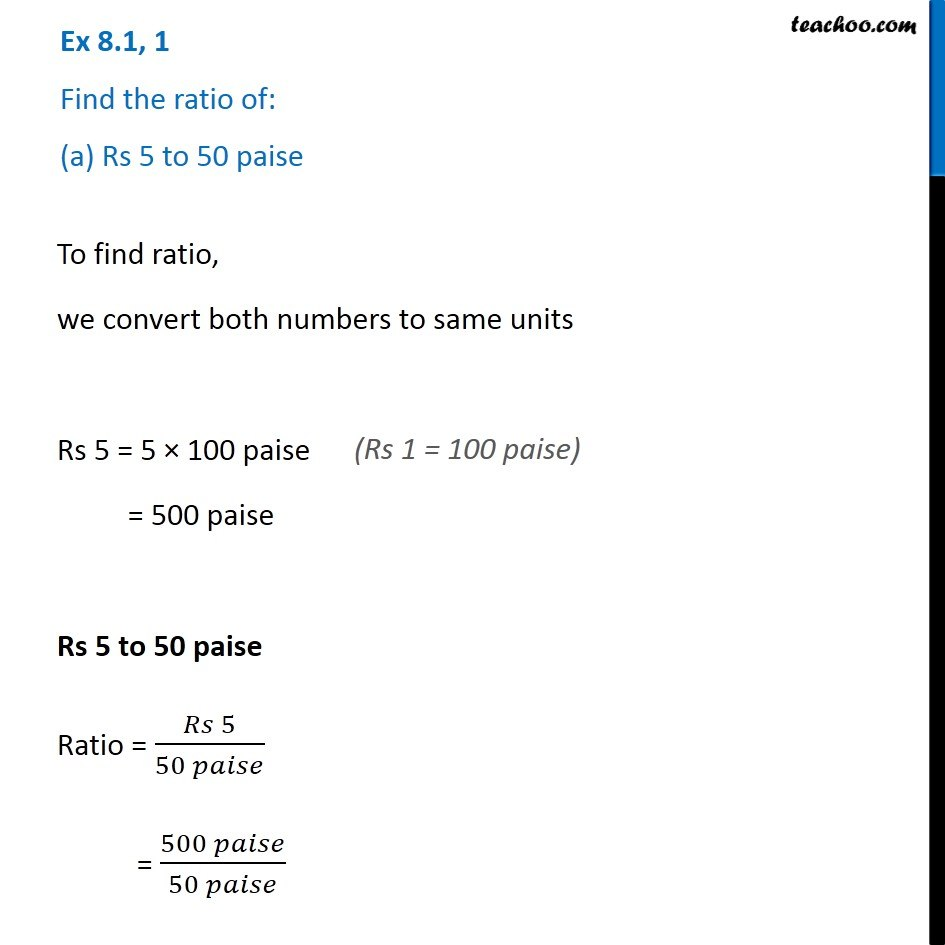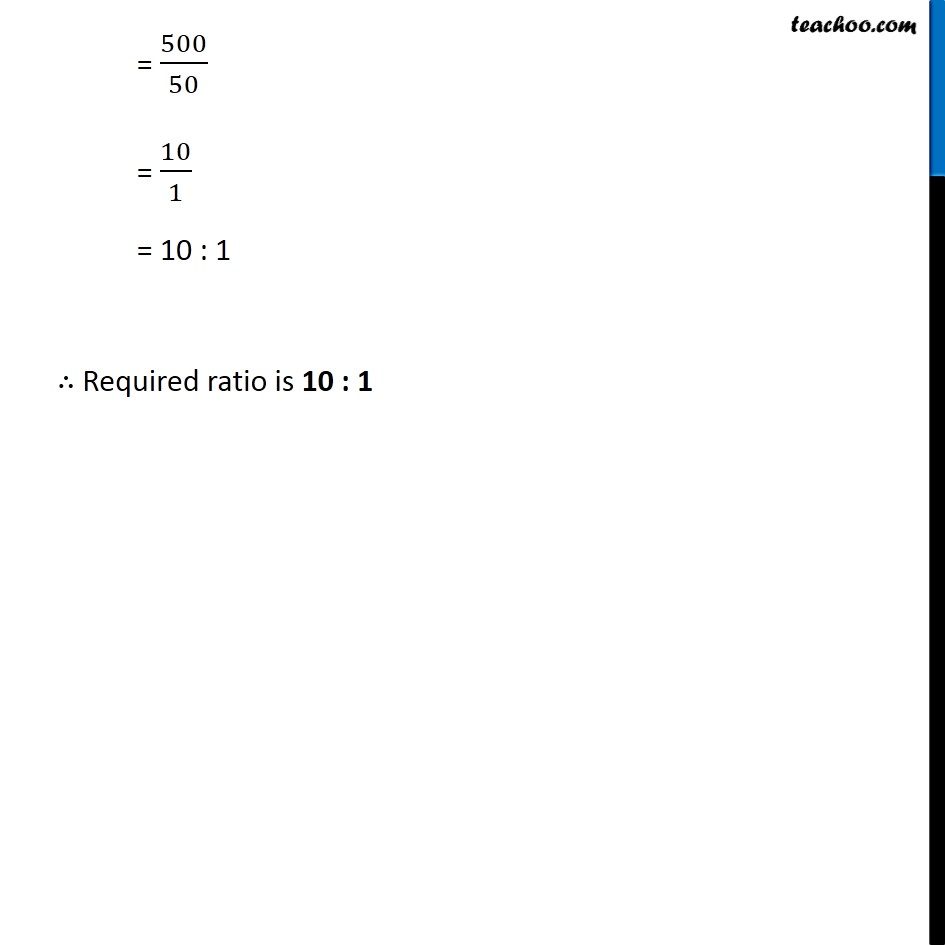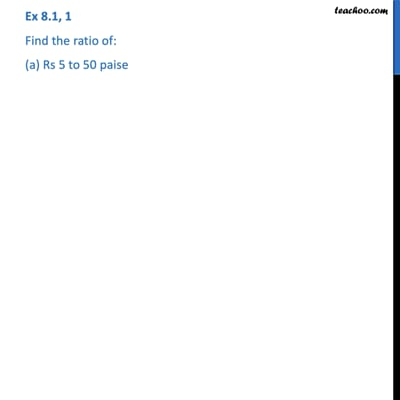Ratios

Chapter 7 Class 7 Comparing Quantities
Concept wiseThis video is only available for Teachoo black users

Learn in your speed, with individual attention - Teachoo Maths 1-on-1 Class

### Transcript

Question 1 Find the ratio of: (a) Rs 5 to 50 paise To find ratio, we convert both numbers to same units Rs 5 = 5 × 100 paise = 500 paise Rs 5 to 50 paise Ratio = (𝑅𝑠 5)/(50 𝑝𝑎𝑖𝑠𝑒) = (500 𝑝𝑎𝑖𝑠𝑒)/(50 𝑝𝑎𝑖𝑠𝑒) = 500/50 = 10/1 = 10 : 1 ∴ Required ratio is 10 : 1